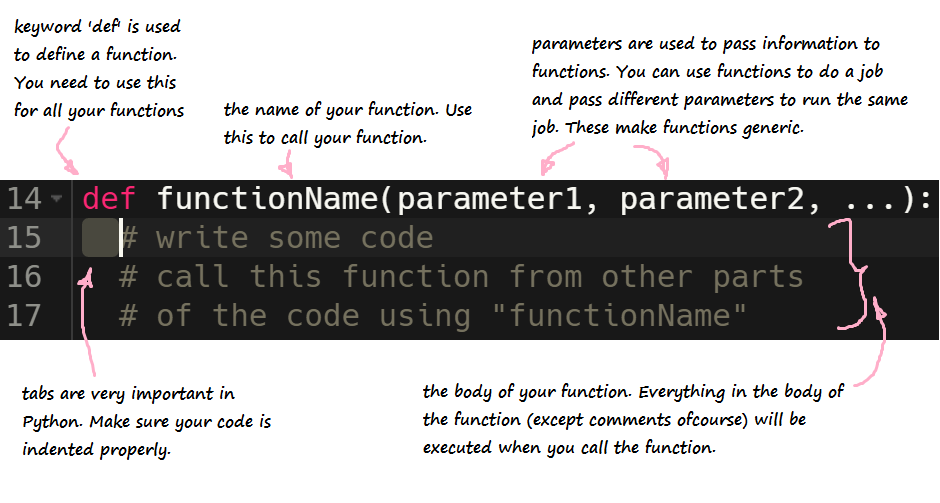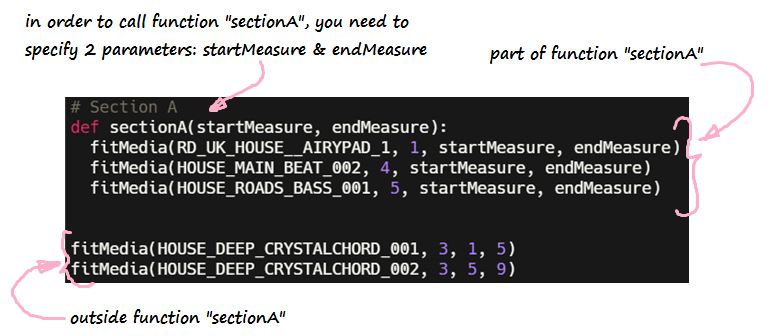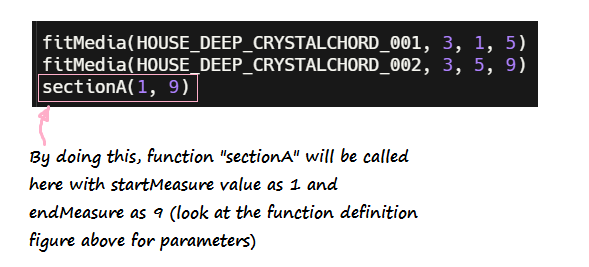# Functions

Functions are a way to organize pieces of similar or repeating code. Rather than having to constantly type out the same code, we can put the desired code in a function and call it whenever we want to use it.

## Creating a function

### Define your function - name & parameters

To make a function in python, we must use the def keyword. This keyword tells the code editor where the function is defined. def is followed by the name of the function. In order to generalize the function (so that we can use it at multiple places), we might need to send some information to it. This is achieved using parameters. They are placed in between parentheses () and follows right after the name of the function. If no parameters are needed, they can be ignored. The function declaration ends with a colon. Here’s what a function looks like:### Write the function body

In Python, indentation defines which code is controlled by the function. For example, look at the following image:The code that is indented underneath the sectionA function is the code that is executed each time function sectionA is called.

## Using the function - Function call

To call a function, input the name of the function and its parameters wherever you want to execute the function. For example, in order to call function sectionA, we would do something like this:### Challenge 1 - Create your own function!

Challenge: Create a function called addNumbers that takes 2 parameters called number1 & number2 and prints the sum on console. Call the function addNumbers with parameters 3 & 5. What is printed on the console?

### Challenge 2

Let’s try to create some simple math functions. Create a function called add that takes in two parameters, number1 and number2, and return the sum of the two numbers.

To check that your function is working correctly, add the following code after your function definition, which calls the function add() and save its value in a variable. For example:

value2 = add(2, 3)
print("This should print 5: " + str(value2))


### Challenge 3

Can you create functions for subtraction, multiplication, and division?

Test the functions out by calling each function, saving its return value, then printing it out to the console.